Custom SearchDERIVATIVE OF CONSTANTS TO VARIABLE POWERS In this section two forms of a constant to a variable power will be presented. The two exponential functions will be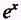and, where x is the variable, a is any constant, and e is equal to 2.71828.... Recalling our study of logarithms in Mathematics, Volume 2-A, since In and e are inverse functions, thenand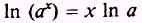If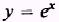then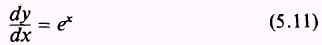PROOF: Since y = In x is differentiable, so is its inverse, y =. To obtain the derivative of y =, we differentiate both sides of equation (5.10) with respect to x, which givesMultiplying both sides of equation (5.12) bygives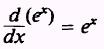Chain rule differentiation and equation (5.11) giveSOLUTION.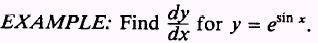SOLUTION.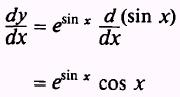IfthenPROOF. Applying logarithmic rules,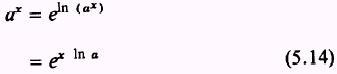Differentiating both sides of equation (5.14) givesNOTE: In a is a constant.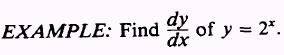SOLUTION:Chain rule differentiation and equation (5.13) give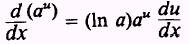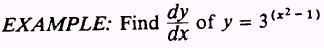SOLUTION: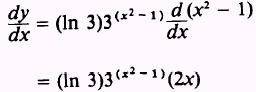PRACTICE PROBLEMS: Find d of the following: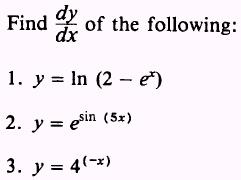ANSWERS: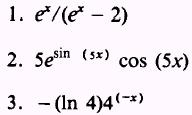SUMMARY The following are the major topics covered in this chapter: 1. Derivative of a constant: Theorem 1. The derivative of a constant is zero.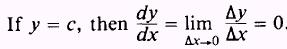2. Derivative of a variable raised to a power: Theorem 2. The derivative of the function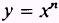is given by, if n is any real number. 3. Derivative of the sum of two or more functions: Theorem 3. The derivative of the sum of two or more differentiable functions of x is equal to the sum of their derivatives. If two functions of x are given, such that u = g(x) and v = h(x), and also y = u + v = g(x) + h(x), then4. Derivative of the product of two or more functions: Theorem 4. The derivative of the product of two differentiable functions of x is equal to the first function multiplied by the derivative of the second function, plus the second function multiplied by the derivative of the first function.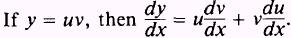This theorem can be extended to three or more functions.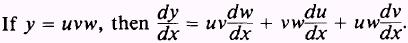5. Derivative of the quotient of two functions: Theorem 5. At a point where the denominator is not equal to zero, the derivative of the quotient of two differentiable functions of x is equal to the denominator times the derivative of the numerator minus the numerator times the derivative of the denominator, all divided by the square of the denominator.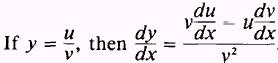6. Derivative of a function raised to a power: Theorem 6. The derivative of any differentiable function of x raised to the power n, where n is any real number, is equal to n times the polynomial function of x to the (n - ])power times the derivative of the polynomial itself. If y =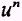, where u is any differentiable function of x, then7. Derivative of a function in radical form: To differentiate a function containing a radical, replace the radical by a fractional exponent; then find the derivative by applying the appropriate theorems. 8. Derivative of a function using the chain rule:where the variable y = f(u) is a differentiable function of u and u = g(x) is differentiable function of x. 9. Derivative of an inverse function: Theorem 7. The derivative of an inverse function is equal to the reciprocal of the derivative of the direct function.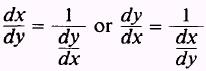10. Derivative of an implicit function: In equations containing x and y, if an equation of y is not solved for, then y is called an implicit function of x. The derivative of each term containing y will be followed by11. Derivative of trigonometric functions:12. Derivative of natural logarithmic functions: Theorem 8. The natural logarithm y = In x has the derivativeIf u is a positive differentiable function of x, then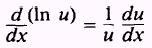13. Derivative of a constant to a variable power: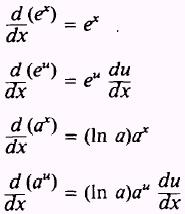where x is a variable, u is a function of x, a is a constant, and e is equal to 2.71828....Integrated Publishing, Inc. - A (SDVOSB) Service Disabled Veteran Owned Small Business